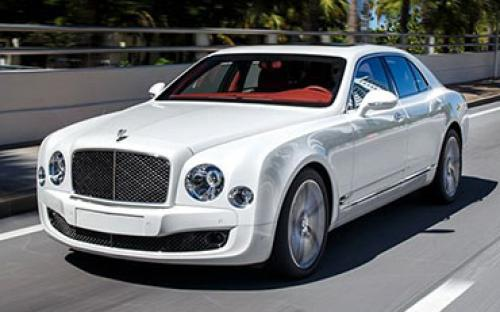### 宾利 慕尚0 种颜色可选2015款最低售价：498.00 万元起

5575(mm)1926(mm)1521(mm)##### 配置亮点：
• 胎压监测装置

• ISOFIX儿童座椅接口

• 车身稳定控制(ESC/ESP/DSC等)

• 电动天窗

• 定速巡航

• 后倒车雷达

• 真皮座椅

• GPS导航系统

• 氙气大灯

• 后视镜加热

• 提交

宾利 慕尚 绕车实拍• 宾利 慕尚 在售车型

排量 车型 厂商指导价 本地最低报价 购车工具
6.8T
6.8T 标准版 8挡手自一体
498.00万
498.00万

6.8T 极致版 8挡手自一体
555.80万
555.80万

### 宾利 慕尚 动力加速

慕尚 0-100公里加速时间分布在 4.9-5.3秒 属于 高性能级

动力级别 加速时间 车型
高性能级(2款)
5.3s
6.8T 标准版
4.9s
6.8T 极致版

宾利 慕尚 视频

宾利 慕尚 新闻资讯

# 宝马2系GranTourer奔驰C级旅行车等多款将上市深港澳车展

车系文章 超过10905次关注

北京时间6月6日上午，2015深港澳车展将正式开幕。在此次深港澳车展上，将有多款新车首发和上市，其中包括了比亚迪唐、宝马2系GranTourer、丰田新埃尔法、奔驰C级旅...

# 2014巴黎车展：宾利慕尚Speed正式发布

新闻 超过4167次关注

在2014巴黎车展上，宾利正式推出了慕尚Speed车型，新车相比普通版慕尚在外观和动力方面均有所变化。

# 售498-555.8万元 新款宾利慕尚上市

进口新车 超过5045次关注

近日，我们获得消息，宾利新款慕尚正式上市，其售价为498.0-555.8万元。详细车型价格见下表。

# 宾利慕尚Speed官图发布 巴黎车展首发

进口新车 超过5708次关注

日前，宾利官方发布了慕尚Speed车型的官图，新车将会在10月开幕的巴黎车展首发。

# 广州车展豪华车跑车 慕尚 幻影特别版

导购 超过4175次关注

慕尚尊贵版在造型设计方面和普通慕尚车型有一些细节区别，比如包围和侧面细节，另外就是为了提升性能而进行的工程技术上调整。另外身为尊贵版车型，配置和豪华感的...

# 中文名首次公布的车型 宾利慕尚

导购 超过3394次关注

宾利新一代旗舰车型Mulsanne亮相北京车展E4馆，中文定名“慕尚”。新车目前可以接受预定，售价600万人民币。它是以法国勒芒赛道的传奇性弯道(Mulsanne)命名。作为近...

# 纯正血统 宾利慕尚用实力证明物有所值

评测 超过5025次关注

跟流水线作业的量产车相比，宾利慕尚的制造速度只能用“漫长”来形容。如果不是在克鲁郡的宾利工厂亲眼目赌它的手工打造过程，我们还是很难直观地明白它之所以昂贵...

# 宾利计划未来90%车型将搭载混合动力系统

新闻 超过3340次关注

近日，宾利官方对外表示将在2020年时完成旗下90%的新车均会推出搭载混动车型的计划，成为宾利车型的主打动力配置。同时官方介绍，届时采用混动系统的车型可提升25%...

# 宾利混合动力概念车将于北京车展首发

新闻 超过3238次关注

日前，宾利官方宣布于即将开幕的北京车展上全球首发旗下全新的混合动力车型。同时宾利也表示该车搭载的混合动力系统也会被用于宾利将在2017年发布的SUV车型上。据悉...

# 宾利慕尚限量版海外上市 全球限量22台

新闻 超过3657次关注

近日，据国外媒体报道，宾利推出了2014款慕尚Birkin限量版车型，用来纪念驾驶宾利赛车参加勒芒大赛并且夺得冠军的“宾利兄弟”。值得注意的是，这款限量车型全球只...

猜你喜欢

﻿
• 快速找车
• 选择品牌
• 选择品牌
• A  奥迪
• A  阿斯顿·马丁
• A  阿尔法·罗密欧
• B  宝沃
• B  布加迪
• B  巴博斯
• B  保时捷
• B  宾利
• B  奔驰
• B  宝马
• B  本田
• B  别克
• B  标致
• B  比亚迪
• B  宝骏
• B  北汽制造
• B  北汽新能源
• B  北汽幻速
• B  北汽威旺
• B  北京汽车
• B  奔腾
• B  北汽绅宝
• C  长安
• C  长安商用
• C  长城
• C  昌河
• D  大众
• D  道奇
• D  DS
• D  东南
• D  东风风神
• D  东风风行
• D  东风小康
• D  东风风度
• D  东风
• F  福特
• F  丰田
• F  菲亚特
• F  法拉利
• F  福田
• F  福迪
• F  福汽启腾
• G  观致
• G  广汽传祺
• G  广汽吉奥
• G  GMC
• H  红旗
• H  汉腾汽车
• H  哈弗
• H  哈飞
• H  海格
• H  海马
• H  华颂
• H  黄海
• H  华泰
• H  恒天
• J  吉利汽车
• J  捷豹
• J  Jeep
• J  江淮
• J  江铃
• J  金杯
• J  九龙
• J  金旅
• K  凯翼
• K  凯迪拉克
• K  克莱斯勒
• K  科尼塞克
• K  卡威
• K  开瑞
• L  路虎
• L  林肯
• L  劳斯莱斯
• L  兰博基尼
• L  雷克萨斯
• L  铃木
• L  雷诺
• L  理念
• L  力帆
• L  莲花汽车
• L  猎豹
• L  路特斯
• L  陆风
• M  马自达
• M  MG
• M  MINI
• M  玛莎拉蒂
• M  摩根
• M  迈凯轮
• N  纳智捷
• O  欧宝
• O  讴歌
• O  欧朗
• Q  奇瑞
• Q  起亚
• Q  启辰
• R  日产
• R  荣威
• R  瑞麒
• S  三菱
• S  斯威汽车
• S  萨博
• S  smart
• S  斯柯达
• S  斯巴鲁
• S  思铭
• S  双龙
• S  上汽大通
• S  双环
• T  特斯拉
• T  腾势
• W  沃尔沃
• W  五菱汽车
• W  五十铃
• W  威兹曼
• W  威麟
• X  现代
• X  雪佛兰
• X  雪铁龙
• X  西雅特
• Y  一汽
• Y  英菲尼迪
• Y  英致
• Y  依维柯
• Y  野马汽车
• Y  永源
• Z  众泰
• Z  中华
• Z  中兴
• Z  知豆
• 选择车系
• 选择车系
• 车型对比
• 选择品牌
• 选择品牌
• A  奥迪
• A  阿斯顿·马丁
• A  阿尔法·罗密欧
• B  宝沃
• B  布加迪
• B  巴博斯
• B  保时捷
• B  宾利
• B  奔驰
• B  宝马
• B  本田
• B  别克
• B  标致
• B  比亚迪
• B  宝骏
• B  北汽制造
• B  北汽新能源
• B  北汽幻速
• B  北汽威旺
• B  北京汽车
• B  奔腾
• B  北汽绅宝
• C  长安
• C  长安商用
• C  长城
• C  昌河
• D  大众
• D  道奇
• D  DS
• D  东南
• D  东风风神
• D  东风风行
• D  东风小康
• D  东风风度
• D  东风
• F  福特
• F  丰田
• F  菲亚特
• F  法拉利
• F  福田
• F  福迪
• F  福汽启腾
• G  观致
• G  广汽传祺
• G  广汽吉奥
• G  GMC
• H  红旗
• H  汉腾汽车
• H  哈弗
• H  哈飞
• H  海格
• H  海马
• H  华颂
• H  黄海
• H  华泰
• H  恒天
• J  吉利汽车
• J  捷豹
• J  Jeep
• J  江淮
• J  江铃
• J  金杯
• J  九龙
• J  金旅
• K  凯翼
• K  凯迪拉克
• K  克莱斯勒
• K  科尼塞克
• K  卡威
• K  开瑞
• L  路虎
• L  林肯
• L  劳斯莱斯
• L  兰博基尼
• L  雷克萨斯
• L  铃木
• L  雷诺
• L  理念
• L  力帆
• L  莲花汽车
• L  猎豹
• L  路特斯
• L  陆风
• M  马自达
• M  MG
• M  MINI
• M  玛莎拉蒂
• M  摩根
• M  迈凯轮
• N  纳智捷
• O  欧宝
• O  讴歌
• O  欧朗
• Q  奇瑞
• Q  起亚
• Q  启辰
• R  日产
• R  荣威
• R  瑞麒
• S  三菱
• S  斯威汽车
• S  萨博
• S  smart
• S  斯柯达
• S  斯巴鲁
• S  思铭
• S  双龙
• S  上汽大通
• S  双环
• T  特斯拉
• T  腾势
• W  沃尔沃
• W  五菱汽车
• W  五十铃
• W  威兹曼
• W  威麟
• X  现代
• X  雪佛兰
• X  雪铁龙
• X  西雅特
• Y  一汽
• Y  英菲尼迪
• Y  英致
• Y  依维柯
• Y  野马汽车
• Y  永源
• Z  众泰
• Z  中华
• Z  中兴
• Z  知豆
• 选择车系
• 选择车系
• 选择车型
• 选择车型
• 意见反馈